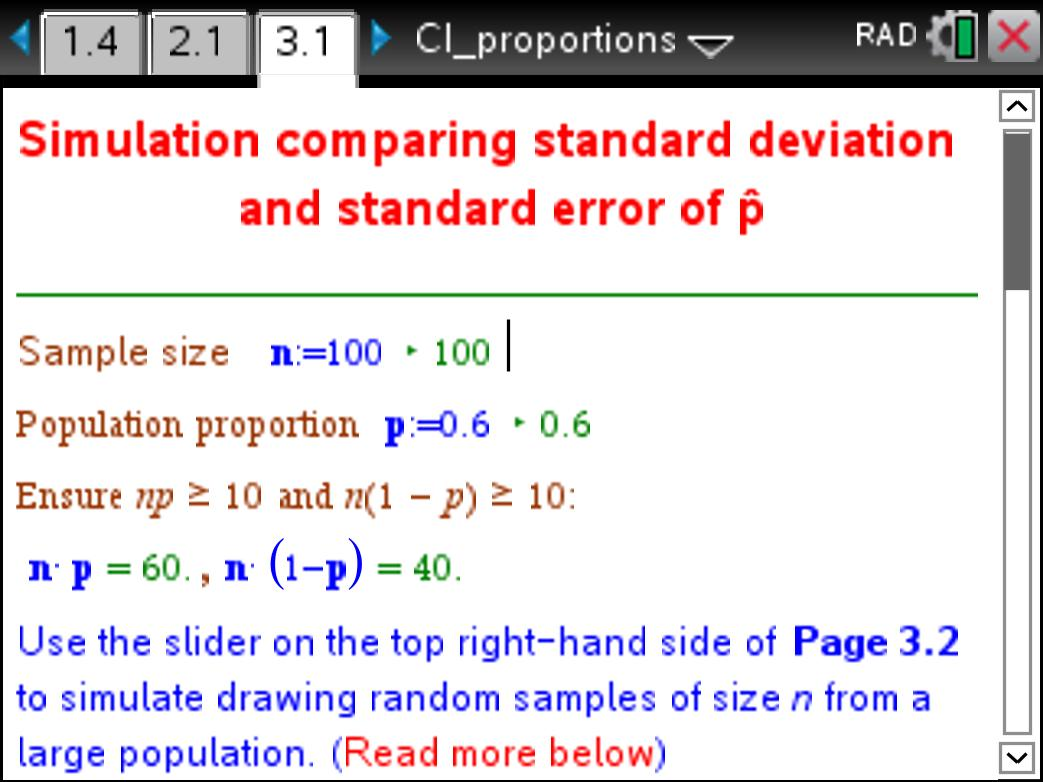# Activities

•• ##### Subject Area

• Standard: VCE: Mathematical Methods: Mathematical Methods

• ##### AuthorAust Senior

60 Minutes

• ##### Device
• TI-Nspire™ CAS
• TI-Nspire™ Navigator™
• ##### Software

TI-Nspire™ CAS
TI-Nspire™ CAS Navigator™ NC System
TI-Nspire™ Navigator™ NC System

4.2

## Confidence intervals for proportions#### Activity Overview

In this activity, the concept of standard error, and the precision of the estimator are developed. Using simulation, students will come to appreciate that an interval estimate can be thought of as a random interval, and will come to an understanding of the significance of a confidence interval.

#### Objectives

• To investigate the precision of the estimator and the concept of standard error.
• To explore the concept of margin of error and confidence intervals for the population proportion .
• To simulate random intervals and come to an understanding of the significance of a confidence interval.

#### Vocabulary

population parameter, population proportion, random sample, sample statistic,
sample proportion, estimator, random variable, sampling distribution, normal
distribution, standard error, margin of error, confidence interval